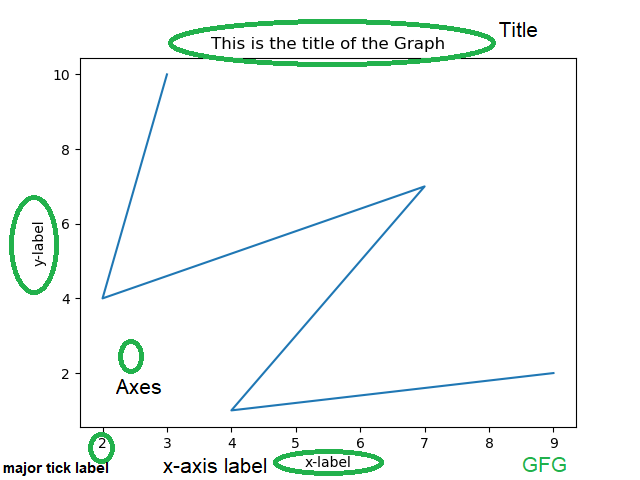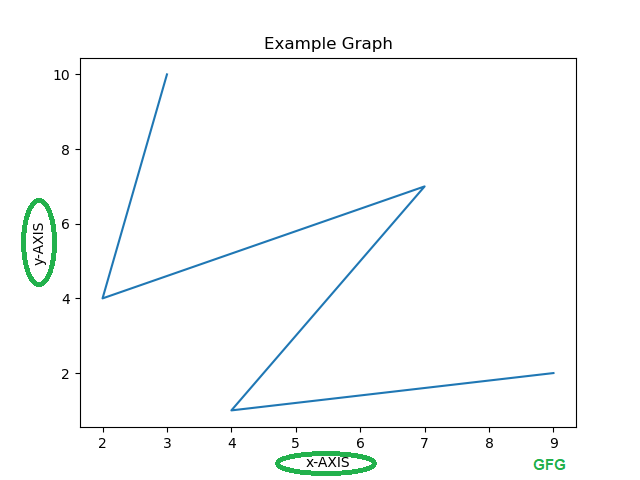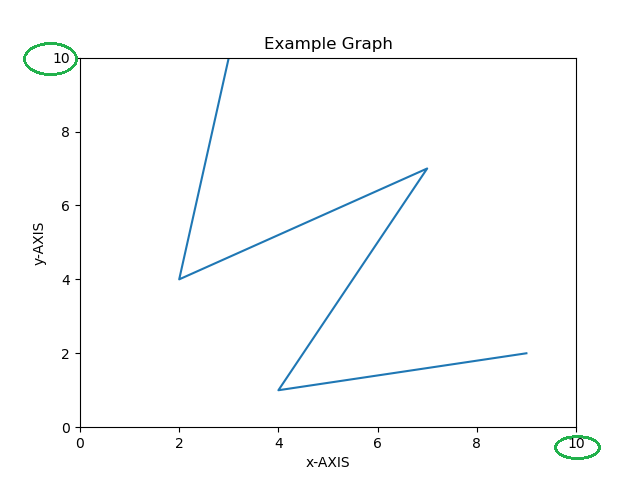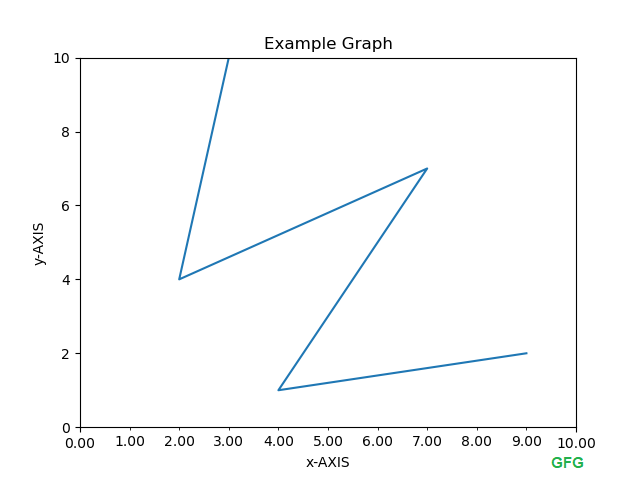Related Articles
Formatting Axes in Python-Matplotlib
• Last Updated : 22 Jun, 2020

Matplotlib is a python library for creating static, animated and interactive data visualizations.

## What is Axes?

This is what you think of as ‘plot’. It is the region of the image that contains the data space. The Axes contains two or three-axis(in case of 3D) objects which take care of the data limits. Below is an image illustrating the different parts of a figure which contains the graph.The different aspects of the Axes can be changed according to the requirements.

1. Labelling x, y-Axis

Syntax:

for x-axis

for y-axis

These functions are used to name the x-axis and y-axis.

Example:

 `# importing matplotlib module``import` `matplotlib.pyplot as plt``import` `numpy as np`` ` `# x-axis & y-axis values``x ``=` `[``3``, ``2``, ``7``, ``4``, ``9``]``y ``=` `[``10``, ``4``, ``7``, ``1``, ``2``]`` ` `# create a figure and axes``fig, ax ``=` `plt.subplots()`` ` `# setting title to graph``ax.set_title(``'Example Graph'``)`` ` `# label x-axis and y-axis``ax.set_ylabel(``'y-AXIS'``)``ax.set_xlabel(``'x-AXIS'``)`` ` `# function to plot and show graph``ax.plot(x, y)``plt.show()`

Output:2. Limits of x, y-Axis

Syntax:

For x-axis:

Axes.set_xlim(self, left=None, right=None, emit=True, auto=False, \*, xmin=None, xmax=None)

Parameters:

• left and right – float, optional
The left xlim(starting point) and right xlim(ending point) in data coordinates. Passing None leaves the limit unchanged.
• auto – bool or None, optional
To turn on autoscaling of the x-axis. True turns on, False turns off (default action), None leaves unchanged.
• xmin, xmax : They are equivalent to left and right respectively, and it is an error to pass both xmin and left or xmax and right.

Returns:
right, left – (float, float)

For y-axis:

Axes.set_ylim(self, bottom=None, top=None, emit=True, auto=False, \*, ymin=None, ymax=None)

Parameters:

• bottom and top – float, optional
The bottom ylim(starting point) and top ylim(ending point) in data coordinates. Passing None leaves the limit unchanged.
• auto – bool or None, optional
To turn on autoscaling of the y-axis. True turns on, False turns off (default action), None leaves unchanged.
• ymin, ymax : They are equivalent to left and right respectively, and it is an error to pass both ymin and left or ymax and right.

Returns:
bottom, top – (float, float)

Example:

 `import` `matplotlib.pyplot as plt``import` `numpy as np`` ` `x ``=` `[``3``, ``2``, ``7``, ``4``, ``9``]``y ``=` `[``10``, ``4``, ``7``, ``1``, ``2``]`` ` `# create a figure and axes``fig, ax ``=` `plt.subplots()`` ` `ax.set_title(``'Example Graph'``)`` ` `ax.set_ylabel(``'y-AXIS'``)``ax.set_xlabel(``'x-AXIS'``)`` ` `# set x, y-axis limits ``ax.set_xlim(``0``, ``10``)``ax.set_ylim(``0``, ``10``)`` ` `# function to plot and show graph``ax.plot(x, y)``plt.show()`

Output:3. Major and Minor Ticks

The Ticks are the values/magnitude of the x and y axis. Minor ticks are divisions of major ticks. There are two classes Locator and Formatter. Locators determine where the ticks are and Formatter controls the formatting of the ticks. These two classes must be imported from matplotlib.

• MultipleLocator() places ticks on multiples of some base.

• FormatStrFormatter uses a format string (e.g., ‘%d’ or ‘%1.2f’ or ‘%1.1f cm’ ) to format the tick labels.

Note: Minor ticks are OFF by default and they can be turned ON by without labels by setting the minor locator and minor tick labels can be turned ON by minor formatter.

Example:

 `# importing matplotlib module and respective classes``import` `matplotlib.pyplot as plt``import` `numpy as np``from` `matplotlib.ticker ``import` `(MultipleLocator,``                               ``FormatStrFormatter,``                               ``AutoMinorLocator)`` ` `x ``=` `[``3``, ``2``, ``7``, ``4``, ``9``]``y ``=` `[``10``, ``4``, ``7``, ``1``, ``2``]`` ` `fig, ax ``=` `plt.subplots()`` ` `ax.set_title(``'Example Graph'``)`` ` `ax.set_ylabel(``'y-AXIS'``)``ax.set_xlabel(``'x-AXIS'``)`` ` `ax.set_xlim(``0``, ``10``)``ax.set_ylim(``0``, ``10``)`` ` `# Make x-axis with major ticks that ``# are multiples of 11 and Label major ``# ticks with '% 1.2f' formatting``ax.xaxis.set_major_locator(MultipleLocator(``10``))``ax.xaxis.set_major_formatter(FormatStrFormatter(``'% 1.2f'``))`` ` `# make x-axis with minor ticks that ``# are multiples of 1 and label minor ``# ticks with '% 1.2f' formatting``ax.xaxis.set_minor_locator(MultipleLocator(``1``))``ax.xaxis.set_minor_formatter(FormatStrFormatter(``'% 1.2f'``))`` ` `ax.plot(x, y)``plt.show()`

Output:Attention geek! Strengthen your foundations with the Python Programming Foundation Course and learn the basics.

To begin with, your interview preparations Enhance your Data Structures concepts with the Python DS Course. And to begin with your Machine Learning Journey, join the Machine Learning – Basic Level Course

My Personal Notes arrow_drop_up